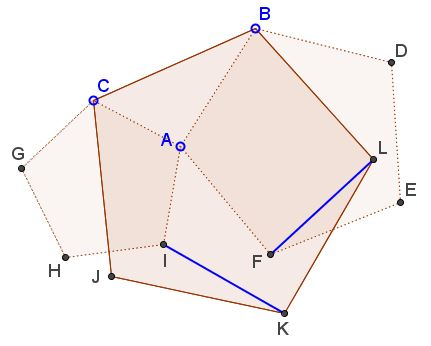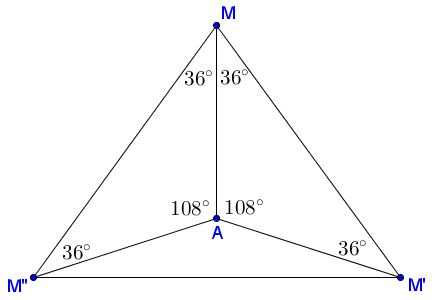# A Problem in Three Pentagons

### Problem

Given three regular pentagons - $ACGHI,$ $ABDEF,$ $BCJKL.$Prove that $FL=IK.$

### Solution

Let $M$ be the center of $BCJKL$ and introduce two rotations, $\sigma$ and $\tau:$ $\sigma$ is the counterclockwise rotation around $M$ through $72^{\circ},$ $\tau$ is a counterclockwise rotation around $A$ through $108^{\circ}.$

Obviously, $I=\tau\sigma\tau F.$Let $M'=\tau^{-1}M,$ $M''=\sigma^{-1}M',$ $M'''=\tau^{-1}M''.$ From the Lemma below we know that $M'''=M.$ However, $\tau\sigma\tau$ is a rotation by

$108^{\circ}+72^{\circ}+108^{\circ}=288^{\circ}=360^{\circ}-72^{\circ}.$

Since it leaves $M$ in place, this is a rotation by $-72^{\circ}$ around $M$ which is $\sigma^{-1}.$

In other words, $F=\sigma I.$ But observe that also $L=\sigma K$ and $K=\sigma J,$ implying that $\sigma(\Delta IJK)=\Delta FKL,$ making the two triangles equal and rotated $72^{\circ}$ relative to each other.

### Lemma

Given an isosceles $\Delta MM'M'',$ with $\angle M'MM''=72^{\circ}.$ Let $A$ be such that $\angle MAM'=\angle M''AM=108^{\circ}.$Then $AM=AM'=AM''.$

### Acknowledgment

The problem which is due to Ruben Dario from the Peru Geometrico group has been communicated to me by Leo Giugiuc along with his solution in complex numbers.